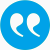#Rudloff, Birgit, Ulus, Firdevs, Vanderbei, Robert. 2017. A parametric simplex algorithm for linear vector optimization problems. Mathematical Programming 163 (1), 213-242.

BibTeX

## Abstract

In this paper, a parametric simplex algorithm for solving linear vector optimization problems (LVOPs) is presented. This algorithm can be seen as a variant of the multi-objective simplex (Evans-Steuer) algorithm . Different from it, the proposed algorithm works in the parameter space and does not aim to find the set of all efficient solutions. Instead, it finds a solution in the sense of Löhne , that is, it finds a subset of efficient solutions that allows to generate the whole frontier. In that sense, it can also be seen as a generalization of the parametric self-dual simplex algorithm, which originally is designed for solving single objective linear optimization problems, and is modified to solve two objective bounded LVOPs with the positive orthant as the ordering cone in Ruszczynski and Vanderbei . The algorithm proposed here works for any dimension, any solid pointed polyhedral ordering cone C and for bounded as well as unbounded problems. Numerical results are provided to compare the proposed algorithm with an objective space based LVOP algorithm (Benson algorithm in ), that also provides a solution in the sense of , and with Evans-Steuer algorithm . The results show that for non-degenerate problems the proposed algorithm outperforms Benson algorithm and is on par with Evan-Steuer algorithm. For highly degenerate problems Benson's algorithm  excels the simplex-type algorithms; however, the parametric simplex algorithm is for these problems computationally much more efficient than Evans-Steuer algorithm.

## Tags

Press 'enter' for creating the tag

## Publication's profile

Status of publication Published WU Journal article Mathematical Programming SCI A FIN-A, INF-A, STRAT-A, WH-A English A parametric simplex algorithm for linear vector optimization problems 163 1 2017 213 242 Y http://arxiv.org/pdf/1507.01895.pdf http://dx.doi.org/10.1007/s10107-016-1061-z

## Associations

People
Rudloff, Birgit (Details)
External
Ulus, Firdevs (Bilkent University, Turkey)
Vanderbei, Robert (Princeton University, United States/USA)
Organization
Institute for Statistics and Mathematics IN (Details)
Research areas (ÖSTAT Classification 'Statistik Austria')
1118 Probability theory (Details)
1137 Financial mathematics (Details)
5361 Financial management (Details)